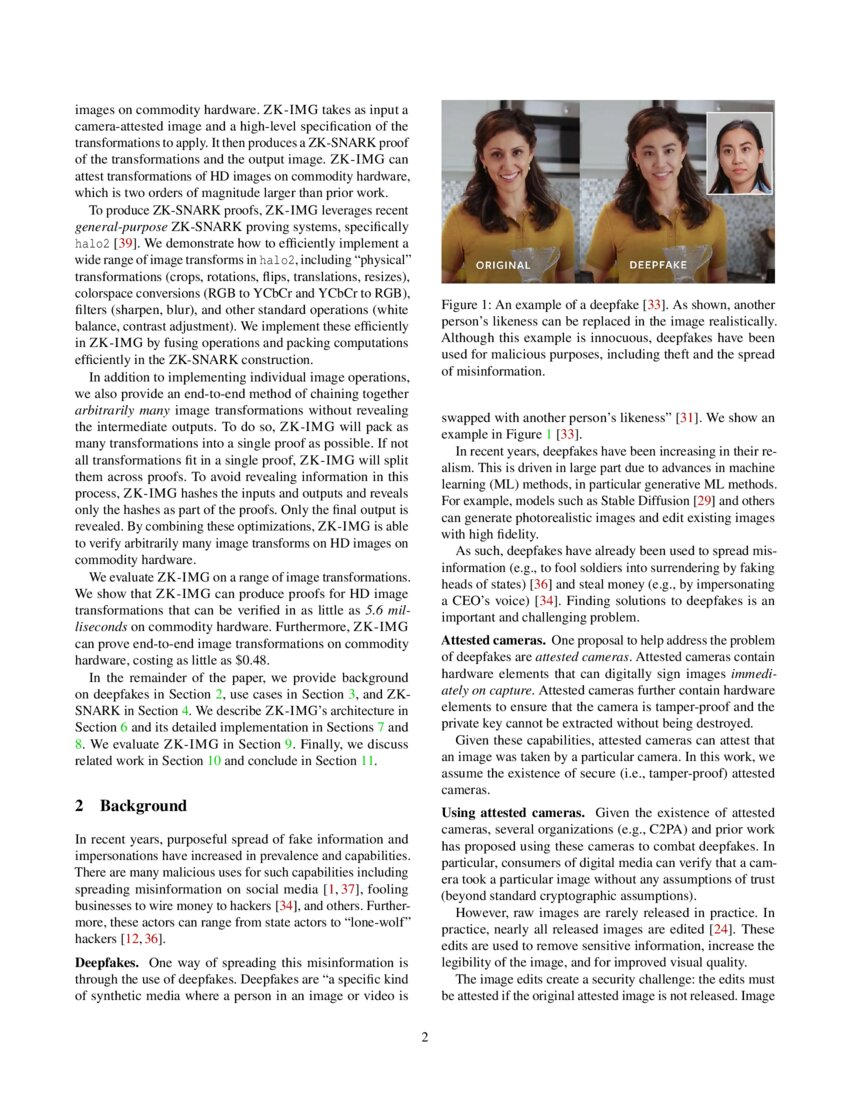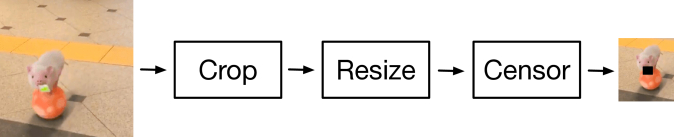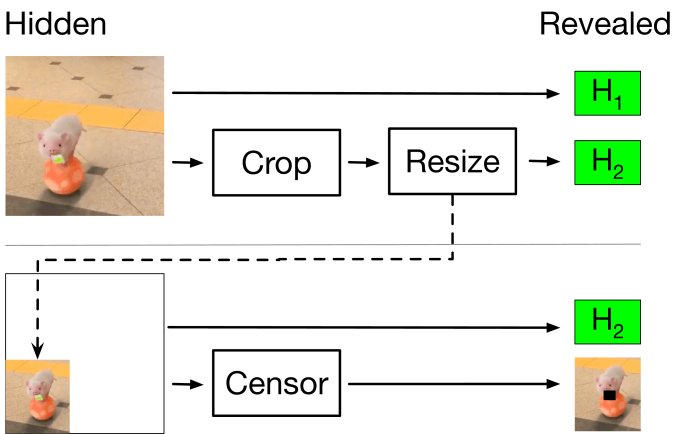# ZK-IMG: Attested Images via Zero-Knowledge Proofs to Fight DisinformationWe first describe how ZK-IMG can produce ZK-SNARK proofs for single image transformations. Although ZK-SNARKs allow arbitrary computations to be proven, we focus on common image transformations that perform “physical” transformations (e.g., crops, rotations) or are the result localized pixel computations (e.g., blurring, colorspace conversions, white balance adjustment).

### 7.1 Common Operations

We now describe how to perform copying, division, and dot products in the Plonkish arithmetization. These operations are used across several image transformations as building blocks.

Copying. One common operation is to copy the value from one cell to another. The Plonkish arithmetization allows copying of cells to be done via the permutation argument. This is easily specified in halo2. Importantly, the set of copied cells (which are constrained to be equal to each other) can be extracted from the verification key.

Division.

Another common operation is integer division with a known, positive divisor. Division is required to do fixed point arithmetic, which approximates floating point arithmetic. As mentioned, the Plonkish arithmetization performs all operations in a large finite field, which does not contain a native division operation.

In order to represent division, we first consider the case of non-negative dividend. Let b=⌊ca⌋. Then, we have that

where 0≤r

Dot products. The last common operation we describe are dot products. Dot products are used for applying convolutional filters as image transformations and also for pixel-level adjustments.

→a and →b, we wish to constrain that c=→a⋅→b. To do so, we can simply have the constraint that

and assign c,ai,bi to 2n+1 cells. If one of the vectors is known ahead of time (e.g., a fixed filter), the values can be omitted and instead hard-coded into the constraint. This reduces the number of assigned cells to n+1 at the cost of adding an additional selector.

Hashes. The halo2 library contains several hash function implementations, which we use. In this work, we assume that the camera produces the Poseidon hash [grassi2019poseidon] when the pixels are packed into the field element. As we show, the hashing is by far the dominant cost of our circuits.

### 7.2 Example Transformations

Given the building blocks we have described, we describe how to use them to construct common image transformations. For brevity, we describe a representative sample of image transformations. The other image transformations in Table 1 can be implemented similarly.

Crop. An image crop takes an image and returns a rectangular subset of the image. Crops can be used to remove sensitive information.

Since a cropped image is a subset of the input image, it can be done entirely through copies. In particular, from an application developer perspective, a crop is equivalent to copying a fixed set of cells. From a verifier perspective, a crop is defined by the set of constraints on the cells.

Image translations, rotations, flips, and nearest neighbor resizing can be done similarly.

Selective removal. A selective removal transformation selectively fills in a fixed set of pixels with black pixels. The fixed set of pixels is typically a rectangle (e.g., a portion of a document) or oval (e.g., a person’s face). The application developer may choose which patterns are permissible. This transformation can be used to censor sensitive parts of the image, such as people’s faces, signs, or documents.

Similar to cropping, the selective removal transformation copies the non-censored pixels from the original image. The censored pixels are copied from a revealed cell that contains the value 0.

Blur. An image blur takes an image and returns an image with a subset of the image with the blur filter applied. Similarly to crops, blurs can be used to remove sensitive information. We describe how to blur the whole image (which is the most resource intensive version), but any subset of the image can be blurred by combining the blurred pixels with copied pixels from the original image.

One common method of applying a blur is to apply a standard Gaussian blur filter, which is a convolutional filter, over the image [shapiro2001computer]. A convolutional filter is a local filter in which pixels nearby the target pixel are combined in a fixed way. Namely,

 y[m,n]=c∑i=−cc∑j=−cx[m−i][n−j]⋅h[i][j].

for a convolutional filter h of size (2c+1)×(2c+1).

To implement the Gaussian blur filter, we perform the following operation per pixel. We perform the unrolled convolution as a dot product and divide the result by the fixed point scalar. The result of the division is the clamped to the valid image range (0 to 255).

Other filters, such as a sharpening filter, can be implemented similarly.

Contrast. A contrast adjustment transformation takes an image and increases or decreases the contrast of the image. Adjusting the contrast of the image can highlight details that may be difficult to see in the unadjusted image.

One common way to adjust the contrast is to adjust each sub-pixel value p by some factor f∈R+ in the following way:

 p′=RoundAndClip(128+f⋅(p−128),0,255)

where RoundAndClip rounds the argument to the nearest integer and clips to 0 to 255 (the standard uint8 range).

To implement the contrast adjustment, we can use a single lookup table. In particular, p takes 256 possible values. Since f is known ahead of time, we can encode the mapping p→p′ with a lookup table.

Other transformations, such as white balance adjustments (which is also a per-sub-pixel map), can be done similarly. Transformations that require full-pixel computations may require additional computation, such as divisions or other constraints.

Color space conversion (RGB to YCbCr). A color space conversion transforms an image from one color space to another. These conversions are often done before applying an image transformation that is more amenable to the converted colorspace. For example, a luminance adjustment requires only adjusting the Y value in the YCbCr colorspace, but requires complex pixel computations in the RGB colorspace. We describe how to convert RGB to YCbCr.

Given the sub-pixel R, G, and B values in RGB color space, we can compute the Y, Cb, and Cr sub-pixel values in YCbCr as follows [hamilton2004jpeg]:

 Y =0 +(0.299⋅ R) +(0.587⋅ G +(0.114⋅ B) Cb =128 −(0.168736⋅ R) −(0.331264⋅ G) +(0.5⋅ B) Cr =128 +(0.5⋅ R) −(0.418688⋅ G) −(0.081312⋅ B)

in floating point. We can convert the floating point operations to fixed point by multiplying all values by a scalar and rounding to the nearest integer. The resulting value can be cast back to the valid range using division as described above. The Y, Cb, and Cr values are rounded to the nearest integer. They need not be clamped due to the range of admissible values.

There are several choices of possible implementations for the RGB to YCbCr color space conversion. One method would be to have a lookup table for the full pixel values. However, this requires a lookup table of size 224, which would force the grid to have at least 225 rows, as several additional rows are required to ensure the zero-knowledge property. Requiring so many rows is resource intensive. Nonetheless, it requires fewer columns (two per pixel).

In many circumstances, it is too resource intensive to have 225 rows. As such, we can implement the RGB to YCbCr color space conversion by explicitly computing the linear functions. Each sub-pixel in YCbCr can be computed as a dot product with known constants and a linear offset (0 or 128). As described above, we can approximate the floating point arithmetic with fixed point arithmetic. Finally, we can divide the results of the fixed point arithmetic by the exponent. Clamping is not necessary as the domain constrains the range to be in 0 to 255.

Other colorspace conversions can be implemented similarly.

Other transformations. As shown in Table 1, we implement several other transformations. These transformations largely follow the pattern of selectively copying pixels and local transformations. Our API allows these patterns to be implemented so they can be easily added and composed.

### 7.3 Optimizations

As described in Section 4, there are several important considerations in the performance of the halo2 proving system and Plonkish arithmetization. We describe several optimizations to improve the performance of the arithmetic circuits. Several similar optimizations were explored in the context of executing machine learning models [kang2022scaling].

Circuit size and packing rows. One of the most critical factors to performance is circuit size. Circuit size is determined by the number of rows and columns in the circuit. As we described in Section 4

, the number of rows must be a power of two. Importantly, for circuit layout, there are sharp phase transitions in circuit size. Furthermore, the memory pressure substantially increases with each power of two.

Naively laying out our circuits would place one operation per row. However, this can be memory inefficient. Namely, for a fixed number of cells, a circuit with fewer rows and more columns is generally more memory efficient.

As such, ZK-IMG will pack as many operations per row as possible. In particular, since the image transformations are largely local pixel computations, ZK-IMG will lay out per-pixel computations and repeat them as many times as possible per row.

For a fixed number of rows, ZK-IMG will optimize the column count by choosing the minimum number of columns needed for the transformations and hashes. ZK-IMG will similarly optimize the number of rows for a fixed number of columns. There is a fixed minimum number of operations required for the hashes, which constrains the minimum number of rows. ZK-IMG

will estimate the total cost of various configurations of rows and columns and pick the minimum cost plan under the memory constraints.

Hard-coding transformation-specific operations. As described above, ZK-IMG provides the verification key in addition to the proof certificate. The verification key encodes the transformations on a per-transformation basis, so application developers need not construct custom arguments for all possible transformation parameters.

In addition to relieving developer effort, per-transformation circuits allow ZK-IMG to perform optimizations. In particular, wherever possible, ZK-IMG will avoid copying values into new cells and will hard-code as many values as possible into custom gates.

As an example of avoiding copies to new cells, consider the operation of a crop, which extracts a subset of an image. Instead of copying the values into new cells in the crop, ZK-IMG can instead return the subset of cells from the input. These cells can subsequently be transformed, hashed, or revealed. This optimization can make intermediate transformations free in terms of prover and verifier cost.

As an example of hard-coding transformation-specific values, consider the blur operation. Instead of laying out the filter values, they can be directly encoded in the custom gate as the constraint. This can save nearly 2× the number of cells, greatly reducing the computational burden.

Sharing lookup tables. A number of operations require lookup tables. For example, they may be used to perform division or clamp a value.

Wherever possible, ZK-IMG will reuse lookup tables. In particular, a common operation is a clamping operation, which performs

 Clamp(x)=Max(Min(x,255),0).

Given the range of possible values of x, we can construct two lookup tables for the input and output respectively. These lookup tables can be shared across transformations that require the clamping operator.

Similarly, ZK-IMG can reuse division remainder range checks for operations that use the same scale factor.(a) Example of a series of image transformations which hides sensitive information (the card in the pig’s mouth).(b) Example of splitting the image transformations across multiple ZK-SNARKs while preserving intermediate transform privacy. Only the hashes (H1,H2) and final image are revealed.

Figure 2: Schematic of ZK-IMG’s procedure to split transformations across ZK-SNARKs while hiding intermediate images.

Source